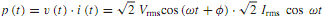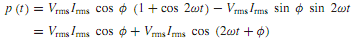## Show power and power factor in ac circuits, Electrical Engineering

Assignment Help:

Q. Show Power and Power Factor in ac Circuits?

Power is the rate of change of energy with respect to time. The unit of power is a watt (W), which is a joule per second (J/s). The use of rms or effective values of voltage and current allows the average power to be found from phasor quantities. Let us consider a circuit consisting of an impedance Z φ = R + jX excited by an applied voltage of v(t) = √2 Vrmscos (ωt + φ), producing a current of i(t) =√2 Irmscosωt . The corresponding voltage and current phasors are then given by V φ and I0°, which satisfy the Ohm's-law relation ¯ V/ ¯ I = V φ/I 0° = Z φ.

The instantaneous power p(t) supplied to the network by the source iswhich can be rearranged as follows by using trigonometric relations:Atypical plot of p(t) is shown in Figure, revealing that it is the sumof an average component, VrmsIrms cos φ, which is a constant that is time independent, and a sinusoidal component, VrmsIrms cos(2ωt +φ), which oscillates at a frequency double that of the original source frequency and has zero average value. The average component represents the electric power delivered to the circuit, whereas the sinusoidal component reveals that the energy is stored over one part of the period and released over another, thereby denoting no net delivery of electric energy. It can be seen that the power p(t) is pulsating in time and its time-average value P is given by Pav = Vrms Irms cos φ since the time-average values of the terms cos 2ωt and sin 2ωt are zero. Note that φ is the angle associated with the impedance, and is also the phase angle between the voltage and the current.

The term cos φ is called the power factor. An inductive circuit, in which the current lags the voltage, is said to have a lagging power factor, whereas a capacitive circuit, in which the current leads the voltage, is said to have a leading power factor. Notice that the power factor associated with a purely resistive load is unity, whereas that of a purely inductive load is zero (lagging) and that of a purely capacitive load is zero (leading).

#### For the given circuit determine voltage, Q. For the circuit shown in Figure...

Q. For the circuit shown in Figure, determine v out (t).

#### Junction in diode, barrier voltage at pn junction

barrier voltage at pn junction

#### The basic operation of battery, The basic operation of battery  A simpl...

The basic operation of battery  A simple cell comprises two dissimilar conductors (electrodes) in an electrolyte. Such a cell comprises copper and zinc electrodes. An electric

#### Explain the bidirectional shift registers, Explain the Bidirectional Shift ...

Explain the Bidirectional Shift Registers? A Reversible or A Bidirectional shift register is one in which the data can be shift either left or right. D flip-flops are used by

Assinment

#### Compute the area of each plate, Q For a parallel-plate capacitor with plate...

Q For a parallel-plate capacitor with plates of area A m 2 and separation d m in air, the capacitance in farads may be computed from the approximate relation Compute the a

#### Show n-channel depletion mode mosfet, Q. For a transistor, when v GS = -2....

Q. For a transistor, when v GS = -2.0 V, find V DSsat if Vp= -4.0 V for an n-channel depletion mode MOSFET. Solution: V p = -(V DSsat + v SG ) but  v SG = -v GS = -(-

#### Determine the slip at maximum torque, A three-phase induction motor, operat...

A three-phase induction motor, operating at its rated voltage and frequency, develops a starting torque of 1.6 times the full-load torque and a maximum torque of 2 times the full-l

#### Subtract contents of memory and borrow from accumulator, Subtract Contents ...

Subtract Contents of Memory and Borrow from Accumulator The contents of the  memory  pointed  by the HL register  pair  and the borrow  flag are subtracted from the  contents

#### Determine the performance of the circuits, With the development of cheap an...

With the development of cheap and reliable operational amplifiers the need to design amplifier circuits has disappeared. However it is still necessary to utilise these devices in a